# Recursion.ppt

30 de Nov de 2022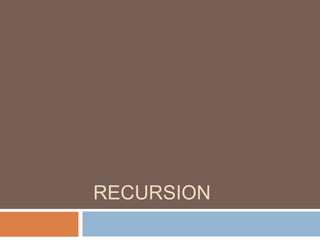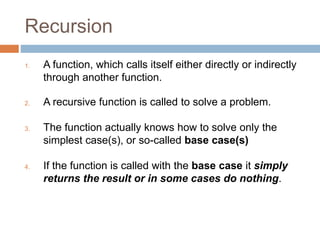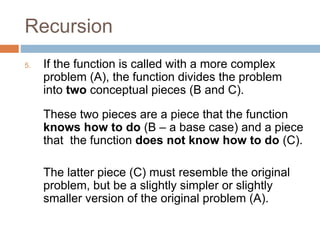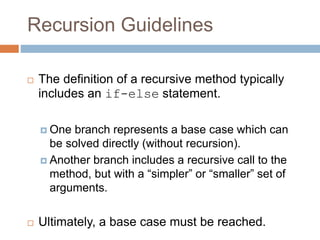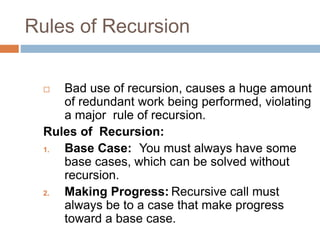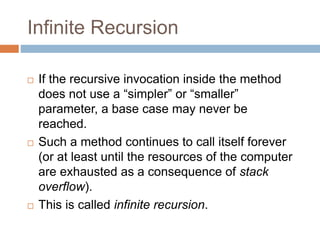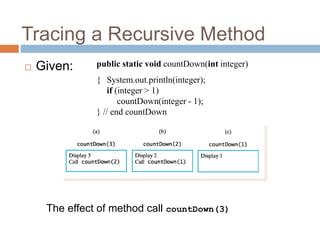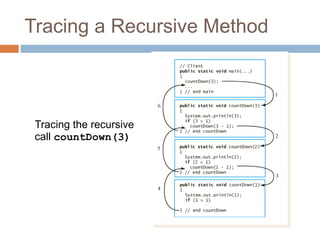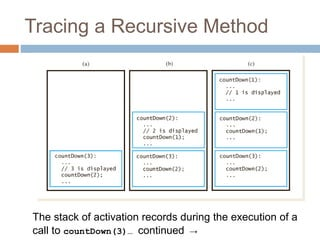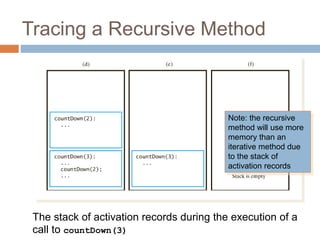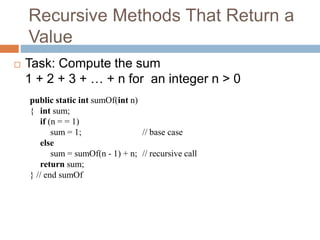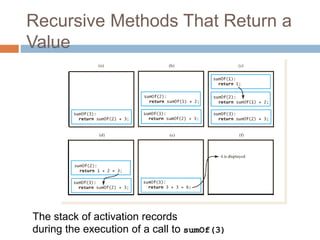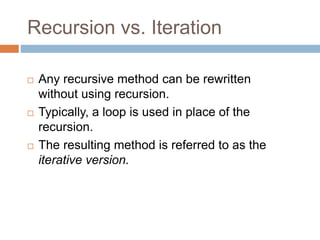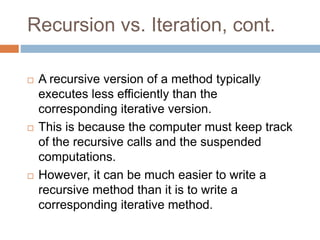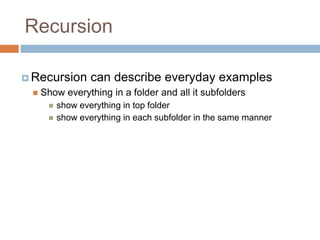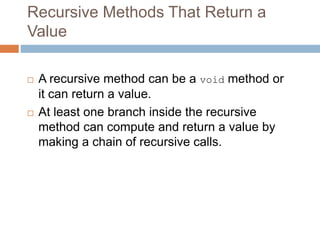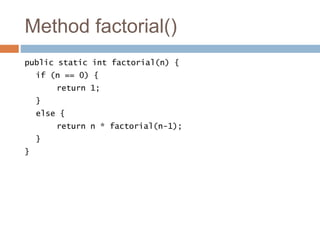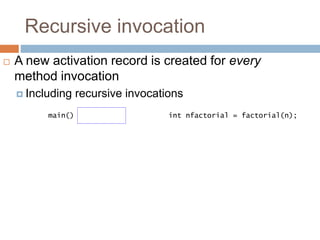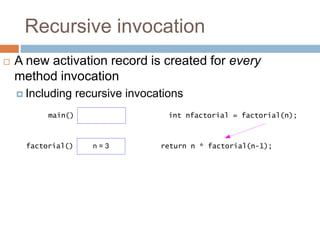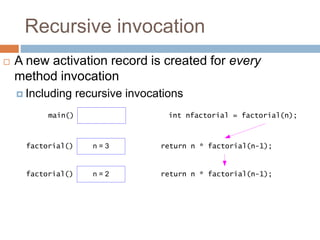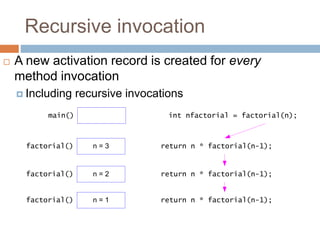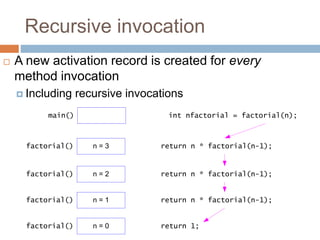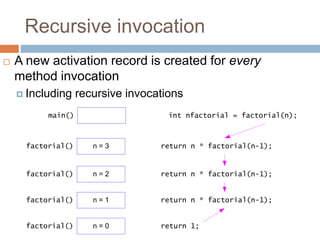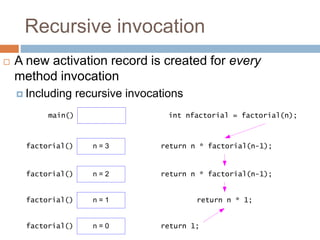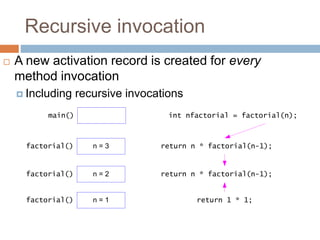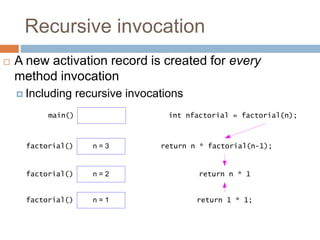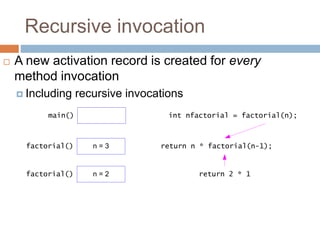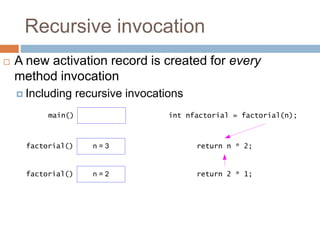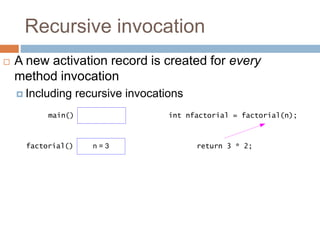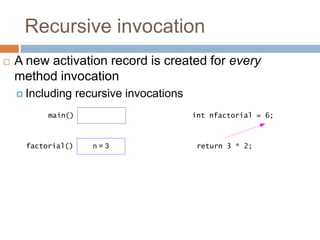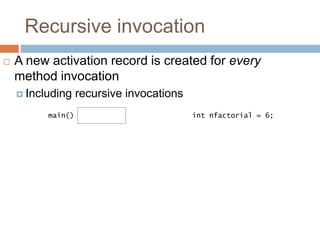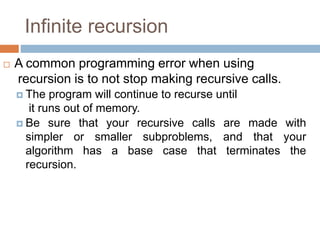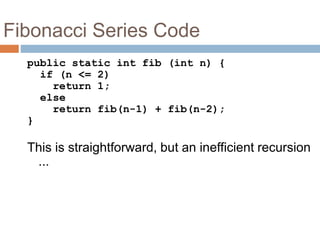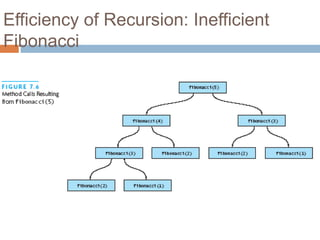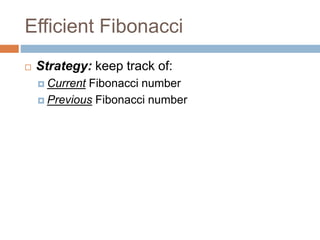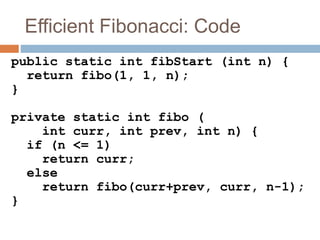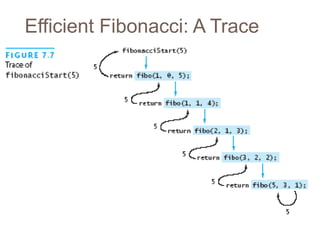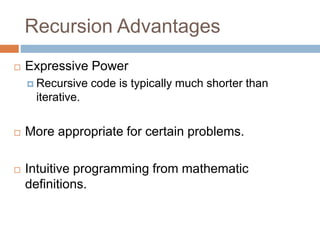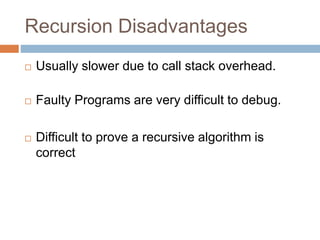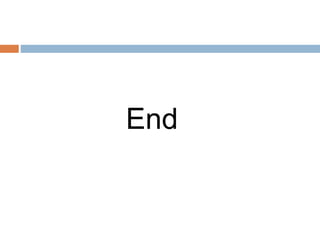1 de 40

### Recursion.ppt

• 2. Recursion 1. A function, which calls itself either directly or indirectly through another function. 2. A recursive function is called to solve a problem. 3. The function actually knows how to solve only the simplest case(s), or so-called base case(s) 4. If the function is called with the base case it simply returns the result or in some cases do nothing.
• 3. Recursion 5. If the function is called with a more complex problem (A), the function divides the problem into two conceptual pieces (B and C). These two pieces are a piece that the function knows how to do (B – a base case) and a piece that the function does not know how to do (C). The latter piece (C) must resemble the original problem, but be a slightly simpler or slightly smaller version of the original problem (A).
• 4. Recursion Guidelines  The definition of a recursive method typically includes an if-else statement.  One branch represents a base case which can be solved directly (without recursion).  Another branch includes a recursive call to the method, but with a “simpler” or “smaller” set of arguments.  Ultimately, a base case must be reached.
• 5. Rules of Recursion  Bad use of recursion, causes a huge amount of redundant work being performed, violating a major rule of recursion. Rules of Recursion: 1. Base Case: You must always have some base cases, which can be solved without recursion. 2. Making Progress: Recursive call must always be to a case that make progress toward a base case.
• 6. Infinite Recursion  If the recursive invocation inside the method does not use a “simpler” or “smaller” parameter, a base case may never be reached.  Such a method continues to call itself forever (or at least until the resources of the computer are exhausted as a consequence of stack overflow).  This is called infinite recursion.
• 7. Tracing a Recursive Method 7  Given: public static void countDown(int integer) { System.out.println(integer); if (integer > 1) countDown(integer - 1); } // end countDown The effect of method call countDown(3)
• 8. Tracing a Recursive Method 8 Tracing the recursive call countDown(3)
• 9. Tracing a Recursive Method 9 The stack of activation records during the execution of a call to countDown(3)… continued →
• 10. Tracing a Recursive Method 10 The stack of activation records during the execution of a call to countDown(3) Note: the recursive method will use more memory than an iterative method due to the stack of activation records
• 11. Recursive Methods That Return a Value 11  Task: Compute the sum 1 + 2 + 3 + … + n for an integer n > 0 public static int sumOf(int n) { int sum; if (n = = 1) sum = 1; // base case else sum = sumOf(n - 1) + n; // recursive call return sum; } // end sumOf
• 12. Recursive Methods That Return a Value 12 The stack of activation records during the execution of a call to sumOf(3)
• 13. Recursion vs. Iteration  Any recursive method can be rewritten without using recursion.  Typically, a loop is used in place of the recursion.  The resulting method is referred to as the iterative version.
• 14. Recursion vs. Iteration, cont.  A recursive version of a method typically executes less efficiently than the corresponding iterative version.  This is because the computer must keep track of the recursive calls and the suspended computations.  However, it can be much easier to write a recursive method than it is to write a corresponding iterative method.
• 15. Recursion  Recursion can describe everyday examples  Show everything in a folder and all it subfolders  show everything in top folder  show everything in each subfolder in the same manner
• 16. Recursive Methods That Return a Value  A recursive method can be a void method or it can return a value.  At least one branch inside the recursive method can compute and return a value by making a chain of recursive calls.
• 17. Method factorial() public static int factorial(n) { if (n == 0) { return 1; } else { return n * factorial(n-1); } }
• 18. Recursive invocation int nfactorial = factorial(n); main()  A new activation record is created for every method invocation  Including recursive invocations
• 19. Recursive invocation int nfactorial = factorial(n); main() return n * factorial(n-1); n = 3 factorial()  A new activation record is created for every method invocation  Including recursive invocations
• 20. Recursive invocation int nfactorial = factorial(n); main() return n * factorial(n-1); n = 3 factorial() return n * factorial(n-1); n = 2 factorial()  A new activation record is created for every method invocation  Including recursive invocations
• 21. Recursive invocation int nfactorial = factorial(n); main() return n * factorial(n-1); n = 3 factorial() return n * factorial(n-1); n = 2 factorial() return n * factorial(n-1); n = 1 factorial()  A new activation record is created for every method invocation  Including recursive invocations
• 22. Recursive invocation int nfactorial = factorial(n); main() return n * factorial(n-1); n = 3 factorial() return n * factorial(n-1); n = 2 factorial() return n * factorial(n-1); n = 1 factorial() return 1; n = 0 factorial()  A new activation record is created for every method invocation  Including recursive invocations
• 23. Recursive invocation int nfactorial = factorial(n); main() return n * factorial(n-1); n = 3 factorial() return n * factorial(n-1); n = 2 factorial() return n * factorial(n-1); n = 1 factorial() return 1; n = 0 factorial()  A new activation record is created for every method invocation  Including recursive invocations
• 24. Recursive invocation int nfactorial = factorial(n); main() return n * factorial(n-1); n = 3 factorial() return n * factorial(n-1); n = 2 factorial() return n * 1; n = 1 factorial() return 1; n = 0 factorial()  A new activation record is created for every method invocation  Including recursive invocations
• 25. Recursive invocation int nfactorial = factorial(n); main() return n * factorial(n-1); n = 3 factorial() return n * factorial(n-1); n = 2 factorial() return 1 * 1; n = 1 factorial()  A new activation record is created for every method invocation  Including recursive invocations
• 26. Recursive invocation int nfactorial = factorial(n); main() return n * factorial(n-1); n = 3 factorial() return n * 1 n = 2 factorial() return 1 * 1; n = 1 factorial()  A new activation record is created for every method invocation  Including recursive invocations
• 27. Recursive invocation int nfactorial = factorial(n); main() return n * factorial(n-1); n = 3 factorial() return 2 * 1 n = 2 factorial()  A new activation record is created for every method invocation  Including recursive invocations
• 28. Recursive invocation int nfactorial = factorial(n); main() return n * 2; n = 3 factorial() return 2 * 1; n = 2 factorial()  A new activation record is created for every method invocation  Including recursive invocations
• 29. Recursive invocation int nfactorial = factorial(n); main() return 3 * 2; n = 3 factorial()  A new activation record is created for every method invocation  Including recursive invocations
• 30. Recursive invocation int nfactorial = 6; main() return 3 * 2; n = 3 factorial()  A new activation record is created for every method invocation  Including recursive invocations
• 31. Recursive invocation int nfactorial = 6; main()  A new activation record is created for every method invocation  Including recursive invocations
• 32. Infinite recursion  A common programming error when using recursion is to not stop making recursive calls.  The program will continue to recurse until it runs out of memory.  Be sure that your recursive calls are made with simpler or smaller subproblems, and that your algorithm has a base case that terminates the recursion.
• 33. Fibonacci Series Code 33 public static int fib (int n) { if (n <= 2) return 1; else return fib(n-1) + fib(n-2); } This is straightforward, but an inefficient recursion ...
• 34. Efficiency of Recursion: Inefficient Fibonacci 34
• 35. Efficient Fibonacci 35  Strategy: keep track of:  Current Fibonacci number  Previous Fibonacci number
• 36. Efficient Fibonacci: Code 36 public static int fibStart (int n) { return fibo(1, 1, n); } private static int fibo ( int curr, int prev, int n) { if (n <= 1) return curr; else return fibo(curr+prev, curr, n-1); }
• 38. Recursion Advantages  Expressive Power  Recursive code is typically much shorter than iterative.  More appropriate for certain problems.  Intuitive programming from mathematic definitions.
• 39. Recursion Disadvantages  Usually slower due to call stack overhead.  Faulty Programs are very difficult to debug.  Difficult to prove a recursive algorithm is correct
• 40. End